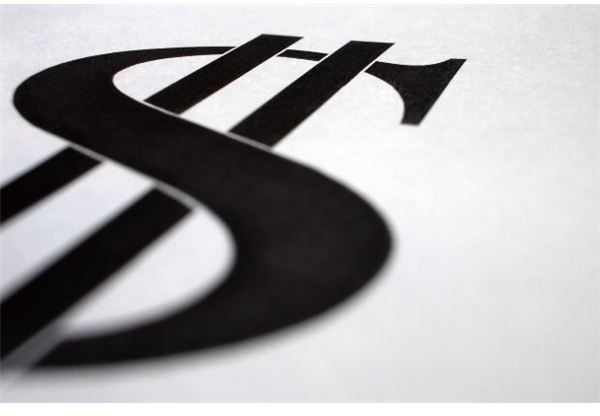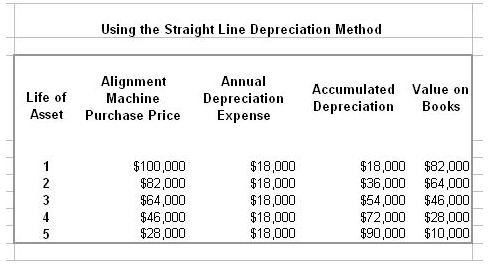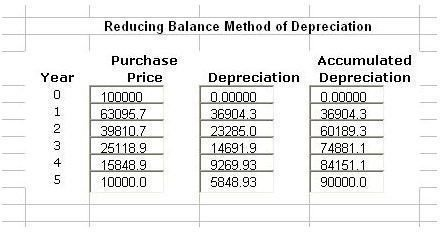# Types of Depreciation Methods: Which One Should You Use?Depreciation is the reduction in the book value of an asset due to usage over a period of time. In other words, it is the reduction in the economic usefulness of the asset, or the calculation of wear and tear that may have occurred to the asset. This is also done to report the actual value to tax authorities.

The present value of the asset, i.e., after deducting the depreciation amount, is then recorded in the accounting books. To calculate, one needs to take into account the economic life and the expected value or scrap value of the asset after its use in the business is over.

The calculation is a non-cash expense that is estimated or forecasted. This process occurs at the end of the financial year and the amount is shown in both the balance sheet and the income and loss statement. Here we discuss the different types of depreciation methods and how to calculate them.

## Straight Line Method

This is the simplest, and most commonly used, form of depreciation calculation and refers to reduction of the value as per a constant rate. The depreciation value is calculated by taking the original, purchase, or historical price, less the scrap (or salvage) value, and dividing it by the useful years or the number of years that the asset would be in use in the business. The rate of depreciation remains constant as a fixed expense throughout the years. This depreciation method is useful for those assets in which the usage remains uniform or consistent. Unfortunately, it does not take into account the fact that all assets do not deteriorate equally.

An example would be an alignment machine purchased for a body shop for \$100,000. The straight line calculation is as follows:

Cost of Alignment Machine – \$100,000

Less Salvage Costs – (\$10,000)

Subtotal – \$90,000

Years of Useful Life – 5

5 Years of Useful Life Divided by \$90,000 = \$18,000

So, for the first year, the alignment machine depreciation using the straight line method is \$18,000 and the value on the accounting books at year end is \$100,000 (purchase price) minus the depreciation (\$18,000) = \$82,000.

The straight line depreciation method continues until the useful life (5 years) of the alignment machine has been reached as seen in the screenshot below (click to enlarge).## Reducing Balance Method

The reducing balance method allows you to consider a certain depreciation percentage to depreciate the alignment machine rate annually. This method takes into consideration an accelerated rate of depreciation. This is useful for those assets in which a higher value is lost during the beginning years of usage. The only flaw of this method is it does not take into account the scrap or residual value of the asset. In addition, most tax professionals will tell you the reducing balance method should be used for fixed assets where useful life is only three years and upon the fourth year, the straight line depreciation method should be implemented (check with your tax professional to be sure). Below is an example of the declining balance method based on five years for our same alignment machine using 37 percent each year.## MACRS Method

The Modified Accelerated Cost Recovery System (MACRS) is widely used in the depreciation of land, buildings or equipment owned and used 100 percent by the company. MACRS is almost always utilized when depreciating assets for tax purposes to enjoy the expense of each depreciated item. In the Bright Hub article, MARCS Depreciation Formula: Explanation and Examples, you'll find great tips on how to use this method to help your business reap the tax benefits offered by this type of depreciation method.

The Internal Revenue Service offers Form 4562 along with detailed instructions on the best way to utilize the MACRS method. You can download the form and instructions here. It you're unsure on how to best utilize the MACRS method, ask your tax professional for help.

## Unit of Production Method

This method refers to an association between the asset’s ability to do work during its useful life and the decline in the worth of the asset. Unfortunately, this depreciation method does not take into account the expected years of the asset but takes into account the measurable units of use. The units could be anything, including number of items produced or hours used for machinery, number of miles traveled by vehicles, etc. Thus, it is calculated by the actual usage of the asset.

Again using our alignment machine and assuming the machine can do 15 alignments in one year (365 days):

Depreciation cost equals original purchase price less salvage value. (\$100,000 – \$10,000 = \$90,000).

Deprecation per item/unit equals depreciable costs divided by the number of total items or units. (\$90,000 / 5,475 units – 16.44%).

Depreciation – \$90,000 * 16.44% = \$14,796 per year.

For additional help on types of depreciation methods, download the free depreciation template from our Media Gallery.

## References

Rhonda Chase, CPA – Telephonic Interview 5/9/2011.

Calculator Soup – https://www.calculatorsoup.com/calculators/financial/depreciation-units-production.php

Dollar Sign – MorgueFile/imelenchon

Screenshots courtesy of author.